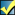A Flash Developer Resource Site

# Thread: [RESOLVED] [CS3] Click then Walk

1. ##[RESOLVED] [CS3] Click then Walk

HelloI'm trying to test myself to make a point & click kind of game. My first try, I managed to make the click thing but i have no idea how to make the walking part XD So it looks like click & appear right nowThe black box is supposed to be unwalkable but I don't know how to do that yet :P

So far, these are the only codes I used, thanks to Flash's Help tab.

Code:
```var mouseListener:Object = new Object();
mouseListener.onMouseDown = function() {
guy._x = _xmouse;
guy._y = _ymouse;
};
Can anyone help?I've attached my .fla file too XDReply With Quote

2. I found out a code and I figured out how to set a constant speed. But the guy shakes when he reaches the target :O Here's what I coded;

Code:
```moveGuy = function () {
this.dx = (this.targx-this._x);
this.dy = (this.targy-this._y);
distance = Math.sqrt(this.dx*this.dx+this.dy*this.dy);
this.vx = (this.dx/distance)*speed;
this.vy = (this.dy/distance)*speed;
this._x += this.vx;
this._y += this.vy;
};

setTarget = function () {
this.targx = _root._xmouse;
this.targy = _root._ymouse;
};

speed = 6;// adjust as required

guy.onEnterFrame = moveGuy;
guy.onMouseDown = setTarget;```Reply With Quote

3. Oh, I think I got itIt works but I'm a bit unsure;

Code:
```moveGuy = function () {
this.dx = (this.targx-this._x);
this.dy = (this.targy-this._y);
distance = Math.sqrt(this.dx*this.dx+this.dy*this.dy);
this.vx = (this.dx/distance)*speed;
this.vy = (this.dy/distance)*speed;
if (distance<4) {
this.vx = 0;
this.vy = 0;
}
this._x += this.vx;
this._y += this.vy;
};

setTarget = function () {
this.targx = _root._xmouse;
this.targy = _root._ymouse;
};

speed = 7;// adjust as required

guy.onEnterFrame = moveGuy;
guy.onMouseDown = setTarget;```
Now how to I make him stop if he gets near the black square?Reply With Quote

4. Hmm, I got him to stop when hits the black wall, but then he won't move after clicking elsewhere :B

Code:
```moveGuy = function () {
trace(distance);
this.dx = (this.targx-this._x);
this.dy = (this.targy-this._y);
distance = Math.sqrt(this.dx*this.dx+this.dy*this.dy);
this.vx = (this.dx/distance)*speed;
this.vy = (this.dy/distance)*speed;
if (this.hitTest(_root.wall) == true) {
this.vx = 0;
this.vy = 0;
} else {
if (distance<4) {
this.vx = 0;
this.vy = 0;
}
}
this._x += this.vx;
this._y += this.vy;
};

setTarget = function () {
this.targx = _root._xmouse;
this.targy = _root._ymouse;
};

speed = 7;// adjust as required

guy.onEnterFrame = moveGuy;
guy.onMouseDown = setTarget;```
Any suggestions?Reply With Quote

5. This didn't work, he keeps going to the upper left or something :O

Code:
```moveGuy = function () {
this.dx = (this.targx-this._x);
this.dy = (this.targy-this._y);
distance = Math.sqrt(this.dx*this.dx+this.dy*this.dy);
this.vx = (this.dx/distance)*speed;
this.vy = (this.dy/distance)*speed;
if (this.hitTest(_root.wall) == true) {
this.vx = 0;
this.vy = 0;
this._x -= 5;
this._y -= 5;
} else {
if (distance<4) {
this.vx = 0;
this.vy = 0;
}
}
this._x += this.vx;
this._y += this.vy;
};

setTarget = function () {
this.targx = _root._xmouse;
this.targy = _root._ymouse;
};

speed = 7;// adjust as required

guy.onEnterFrame = moveGuy;
guy.onMouseDown = setTarget;```Reply With Quote

6. I found this nice point & click walking method: http://board.flashkit.com/board/showthread.php?t=767920

His characters can only walk on a certain pathway. How do I do this? I've tried looking at his .fla but my beginner's knowledge can't decipher it XD It's also in Spanish :P

Pls, helpReply With Quote

7. Well, it took me awhile but I think the best way to make this work is using walls to block the guy's pathI've added something so you all can test this out. You can turn the walls on and off. When you reach the door, someone will tell you to go awayI'm happy with this, so thanks for those who've helpedClick & WalkReply With Quote

####Posting Permissions

• You may not post new threads
• You may not post replies
• You may not post attachments
• You may not edit your posts
•

 » Home » Movies » Tutorials » Submissions » Board » Links » Reviews » Feedback » Gallery » Fonts » The Lounge » Sound Loops » Sound FX » About FK » Sitemap